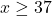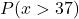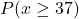## Consider the probability that no less than 37 out of 295 cell phone calls will be disconnected. Choose the best description of the area und

Question

Consider the probability that no less than 37 out of 295 cell phone calls will be disconnected. Choose the best description of the area under the normal curve that would be used to approximate binomial probability.

a. Area to the right of 36.5
b. Area to the right of 37.5
c. Area to the left of 36.5
d. Area to the left of 37.5
e. Area between 36.5 and 37.5

in progress 0
6 months 2021-07-23T09:16:50+00:00 1 Answers 9 views 0

## Answers ( )

1. ### Answer:  Choice A) Area to the right of 36.5

==========================================================

Explanation:

The phrasing “no less than” means the same as “at least”.

Saying “at least 37” means 37 is the lowest we can go.

If x is the number of disconnected calls, thenand we want to find the probability of this happening (the max being 295).

We could use the binomial distribution to find the answer, but that would require adding 295-37+1 = 259 different values which could get tedious. So we could use the normal approximation to make things relatively straight forward.

Assuming this binomial meets the requirements of the normal approximation, then we’d look under the normal curve for the area to the right of 36.5; which is why the answer is choice A.

Why 36.5 and not 37? This has to do with the continuity correction factor when translating from a discrete distribution (binomial) to a continuous one (normal).

If we used 37, then we’d be missing out on the edge case. So we go a bit beyond 37 to capture 36.5 instead. It’s like a fail safe to ensure we do account for that endpoint of 37. It’s like adding a buffer or padding.

————

Side notes:

• Choice B would be the answer if we wanted to excluded 37 from the group, ie if we wanted to calculateinstead of. So we’re moving in the opposite direction of choice A to avoid that edge case.
• We go with “right” instead of “left” since this is what the inequality sign says.